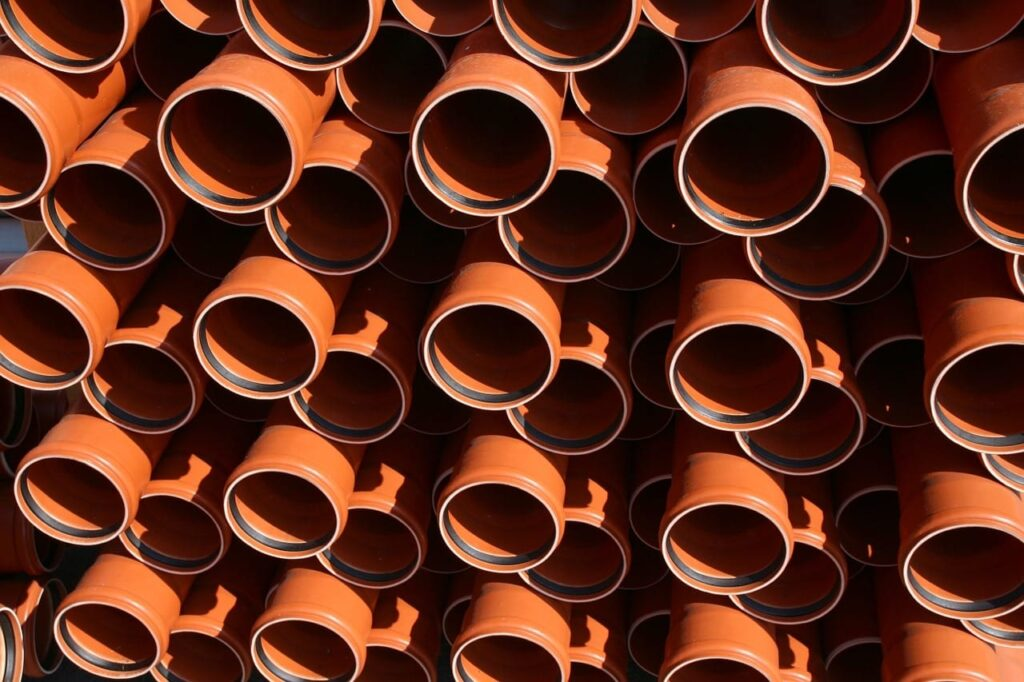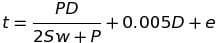# How To: Calculate The Minimum Required Wall Thickness For Tubing

In ASME code calculations involving cylindrical components for 3rd Class Power Engineering you’ll be required to know How To Calculate The Minimum Required Wall Thickness For Tubing. The tubing you may be required to determine the minimum thickness of could be but not limited to superheater tubes or water tube boiler tubes. This post helps explain the material covered in:

All referenced page numbers below are from the 2007 ASME Boiler & Pressure Vessel Code.

## Formula

To determine the minimum required thickness of tubing you will use a formula contained within ASME Boiler & Pressure Vessel Code PG-27 Cylindrical Components Under Internal Pressure. Specifically, PG-27.2.1 on page 8.

PG-27.2.1 Tubing- Up to and including 5in. (125mm) outside diameter. It is important to remember this point as any piece of material with an outside diameter bigger then 125mm is now considered to be piping and the equation provided in PG-27.2.2 page 10 must be used.

Formula to calculate the minimum required thickness:## Formula Variables

The symbols used within the PG-27 formulas are contained within paragraph PG-27.3 page 10 and are defined as follows.

C = Minimum allowance for threading and structural stability (mm) (PG-27.4, note 3) page 11

D = Or O.D. is Outside diameter of cylinder (mm) “In this case tubing”

E = Efficiency of longitudinal welded joints or of ligaments between openings, whichever is lower (the values allowed for E are listed in PG-27.4, note 1) page 11

e = Thickness factor for expanded tube ends (mm) (see PG-27.4, note 4) page 11

P = Maximum allowable working pressure “Gauge Pressure” (MPa) (see PG-21, refers to gauge pressure)

R = Inside radius of cylinder (mm) “In this case tubing”

S = Maximum allowable stress value at the operating temperature of the metal (Section II, Part D, Table 1A. See PG-27.4, note 2) page 11. It is important when determining the maximum allowable stress value to check (PG-6 plate materials) page 4 and (PG-9 boiler tube materials) page 5 before starting calculations as this information will determine the correct stress table to use by indicating whether the material is carbon steel or an alloy steel.

t = minimum required thickness (mm) (see PG-27.4, note 7) page 12

y = temperature coefficient (see PG-27.4, note 6) page 11

## How To Calculate The Minimum Required Wall Thickness For Tubing

Note: All code questions are to be calculated in (mm) and (MPa) unless otherwise stated. Convert accordingly and properly before the calculation.

How To Determine The Minimum Required Wall Thickness For Tubing Practice Question #1

How To Determine The Minimum Required Wall Thickness For Tubing Practice Question #2

## Summary

Hopefully the examples provided help you understand how to calculate the minimum required wall thickness for tubing. If you have additional questions, feedback, or ideas to improve the content provided please let me know in the comment section below.

Power Engineering 101

### 1 thought on “How To: Calculate The Minimum Required Wall Thickness For Tubing”

1.how to know the value of e in tube wall thickness